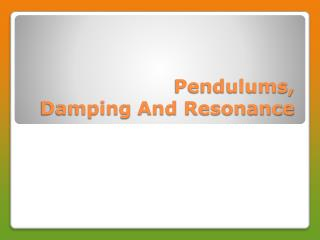DownloadDownload PresentationPendulums, Damping And Resonance

# Pendulums, Damping And Resonance

Download Presentation## Pendulums, Damping And Resonance

- - - - - - - - - - - - - - - - - - - - - - - - - - - E N D - - - - - - - - - - - - - - - - - - - - - - - - - - -
##### Presentation Transcript

1. Pendulums, Damping And Resonance

2. Small object (the bob) suspended from the end of a lightweight cord • Motion of pendulum very close to SHM if the amplitude of oscillation is fairly small • Restoring force is the component of the bobs weight – depends on the weight and the angle Pendulum

3. T = 2√(L/ g) • Period does not depend on the mass • Period does not depend on the amplitude Period of Pendulum

4. Estimate the length of the pendulum in a grandfather clock that ticks once every second. B) what would the period of a clock with a 1.0m length be? Example

5. Will a grandfather clock keep the same time everywhere? What will a clock be off if taken to the moon where gravity is 1/6 that of the earth’s? Example

6. A breeze sets into oscillation a lamp suspended from the ceiling. If the period is 1.0sec, what is the distance from the ceiling to the lamp? • A pendulum can be used to determine the value of g. If the period of a pendulum of length 36.90cm is found to be 1.220 sec, what is the experimental value of g? Practice

7. In any real oscillating system, the amplitude of the oscillations decreases in time until eventually stopping altogether • Effect is called damping • Generally due to air resistance or internal friction • Energy dissipated to thermal energy over time Damping

8. Damping does alter the frequency slightly, but not enough to matter in SHM equations Effects of Damping

9. Every vibrating system has its own natural frequency • If you try to vibrate the system at other than its natural frequency, it will bounce around but never reach any great amplitude • For example, if you push on a swing at random frequency Resonance

10. If you push on the object at the same frequency as its natural frequency, the amplitude will increase dramatically • This effect is called resonance • The natural vibrating frequency of an object is its resonant frequency

11. Music note played at same frequency as the natural vibrating frequency of a crystal glass will cause a forced vibration of the crystal • If resulting vibration is great enough in amplitude that it exceeds the glass’s elastic limit, the glass shatters Breaking Glass